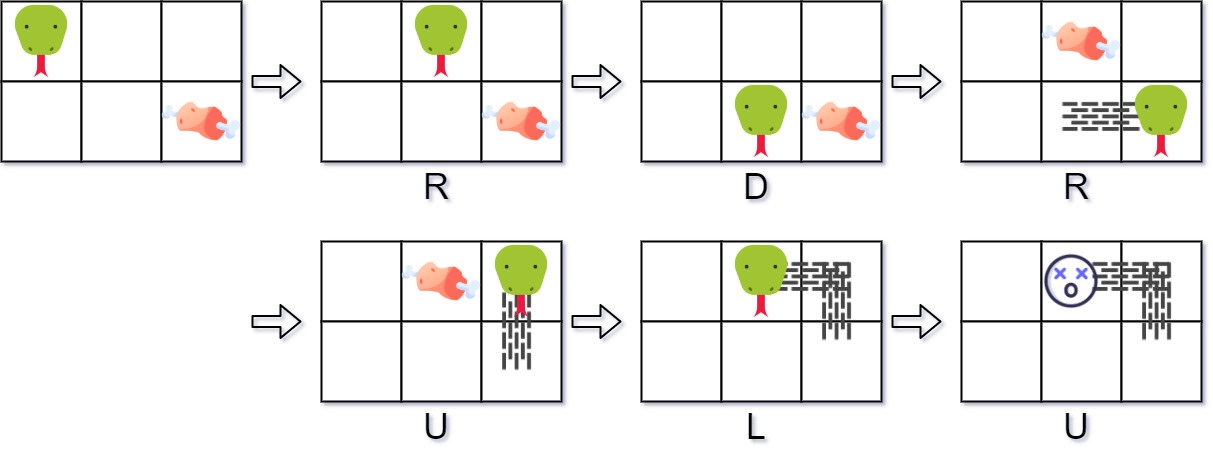353. Design Snake Game

Design a Snake game that is played on a device with screen size `height x width`. Play the game online if you are not familiar with the game.

The snake is initially positioned at the top left corner `(0, 0)` with a length of `1` unit.

You are given an array `food` where `food[i] = (ri, ci)` is the row and column position of a piece of food that the snake can eat. When a snake eats a piece of food, its length and the game's score both increase by `1`.

Each piece of food appears one by one on the screen, meaning the second piece of food will not appear until the snake eats the first piece of food.

When a piece of food appears on the screen, it is guaranteed that it will not appear on a block occupied by the snake.

The game is over if the snake goes out of bounds (hits a wall) or if its head occupies a space that its body occupies after moving (i.e. a snake of length 4 cannot run into itself).

Implement the `SnakeGame` class:

• `SnakeGame(int width, int height, int[][] food)` Initializes the object with a screen of size `height x width` and the positions of the `food`.
• `int move(String direction)` Returns the score of the game after applying one `direction` move by the snake. If the game is over, return `-1`.

Example 1:```Input
["SnakeGame", "move", "move", "move", "move", "move", "move"]
[[3, 2, [[1, 2], [0, 1]]], ["R"], ["D"], ["R"], ["U"], ["L"], ["U"]]
Output
[null, 0, 0, 1, 1, 2, -1]

Explanation
SnakeGame snakeGame = new SnakeGame(3, 2, [[1, 2], [0, 1]]);
snakeGame.move("R"); // return 0
snakeGame.move("D"); // return 0
snakeGame.move("R"); // return 1, snake eats the first piece of food. The second piece of food appears
// at (0, 1).
snakeGame.move("U"); // return 1
snakeGame.move("L"); // return 2, snake eats the second food. No more food appears.
snakeGame.move("U"); // return -1, game over because snake collides with border
```

Constraints:

• `1 <= width, height <= 104`
• `1 <= food.length <= 50`
• `food[i].length == 2`
• `0 <= ri < height`
• `0 <= ci < width`
• `direction.length == 1`
• `direction` is `'U'`, `'D'`, `'L'`, or `'R'`.
• At most `104` calls will be made to `move`.

353. Design Snake Game
``````use std::collections::HashSet;
use std::collections::VecDeque;

#[derive(PartialEq, Eq, Clone, Copy, Hash)]
struct Point(i32, i32);

impl Point {
fn from_direction(direction: String) -> Point {
if direction == "U" {
Point(-1, 0)
} else if direction == "L" {
Point(0, -1)
} else if direction == "R" {
Point(0, 1)
} else if direction == "D" {
Point(1, 0)
} else {
Point(0, 0)
}
}
}

struct SnakeGame {
body: VecDeque<Point>,
food: Vec<Point>,
screen: HashSet<Point>,
n: i32,
m: i32,
score: i32,
}

impl SnakeGame {
fn new(width: i32, height: i32, food: Vec<Vec<i32>>) -> Self {
let score = 0;
let n = height as i32;
let m = width as i32;
let mut body: VecDeque<Point> = VecDeque::new();
let mut screen: HashSet<Point> = HashSet::new();
screen.insert(Point(0, 0));
let food = food.iter().rev().map(|v| Point(v, v)).collect();
SnakeGame {
body,
food,
screen,
n,
m,
score,
}
}

fn make_a_move(&mut self, direction: String) -> i32 {
if self.score == -1 {
return -1;
}
let offset = Point::from_direction(direction);

let tail = self.body.pop_back().unwrap();
self.screen.remove(&tail);

if next.0 < 0
|| next.0 >= self.n
|| next.1 < 0
|| next.1 >= self.m
|| self.screen.contains(&next)
{
return -1;
}
self.body.push_front(next);
self.screen.insert(next);

if let Some(food) = self.food.last() {
if *food == next {
self.food.pop();
self.score += 1;
self.screen.insert(tail);
self.body.push_back(tail);
}
}
self.score
}
}

#[test]
fn test() {
let mut snake = SnakeGame::new(3, 2, vec_vec_i32![[1, 2], [0, 1]]);
assert_eq!(snake.make_a_move("R".to_string()), 0);
assert_eq!(snake.make_a_move("D".to_string()), 0);
assert_eq!(snake.make_a_move("R".to_string()), 1);
assert_eq!(snake.make_a_move("U".to_string()), 1);
assert_eq!(snake.make_a_move("L".to_string()), 2);
assert_eq!(snake.make_a_move("U".to_string()), -1);
}
``````